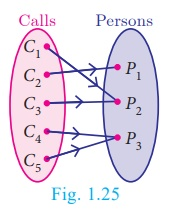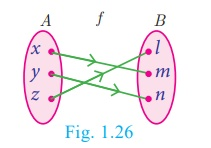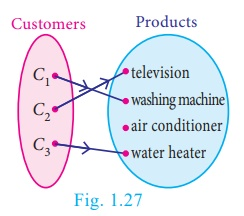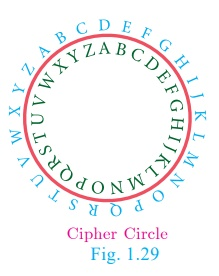Home | | Maths 10th Std | Types of Functions

# Types of Functions

In this section, we will discuss the following types of functions with suitable examples. (i) one – one (ii) many – one (iii) many – one (iv) into

Types of Functions

In this section, we will discuss the following types of functions with suitable examples.

(i) one – one

(ii) many – one

(iii) many – one

(iv) into

### 1. One – one function

Let us assume that we have a cell phone with proper working condition. If you make a usual call to your friend then you can make only one call at a time (Fig.1.21).If we treat making calls as a function, then it will be one – one.

A function f : AB is called one – one function if distinct elements of A have distinct images in B.

A one-one function is also called an injection.

Equivalently,

If for all a1, a2 A , f (a1) = f (a2 ) implies a1 = a2 , then f is called one – one function.

Illustration 10

A = {1,2,3,4} and B = {a,b,c,d,e}

(i) Let f = {(1,a), (2,b), (3,d), (4,c)}

In Fig. 1.22, for different elements in A, there are different images in B.

Hence f is a one – one function.(ii) Let g = {(1,a), (2,b), (3,c), (4,e)}

g is a function from A to  such  that  g(1) = g(2) = b , but 1 ≠ 2.

Thus two distinct elements 1 and 2 in the first set A have same image b the second set in B (Fig.1.23). Hence, g is not a one–one function.### 2. Many – one function

In a theatre complex three films F1, F2 , F3 are shown. Seven  persons (P1 to P 7) arrive at the theatre and buy tickets as shown (Fig 1.24).If the selection of films is  considered as  a  relation, then this  is a function which is many–one, since more than one person may choose to watch the same film.

A function f : A B is called many-one function if two or more elements of A have same image in B.

In other words, a function f: AB is called many-one if f it is not one–one.

Illustration 11

Let A = {1,2,3,4} and ; Let f = {(1,a), (2,a), (3,b), (4,c)}

Then f is a function from A to B in which different elements 1 and 2 of A have the same image a in B. Hence f is a many – one function.

### 3. Onto function

In a mobile phone assume that there are 3 persons in the contact. If every person in the contact receives a call, then the function representing making calls will be onto. (Fig.1.25)A function f : A B is said to be onto function if the range of f is equal to the co-domain of f.

In other words, every element in the co-domain B has a pre-image in the domain A. An onto function is also called a surjection.

note

If f : A → B is an onto function then, the range of f = B . That is, f (A) = B .

Illustration 12

Let A = {x,y, z}, B = {l,m,n} ;

Range of f = {l,m,n} = (Fig.1.26)Hence f is an onto function.

### 4. Into function

In a home appliance showroom, the products television, air conditioner, washing machine and water heater were provided with 20% discount as new year sale offer. If the selection of the above products by the three customers C1, C2, C3 is considered as a function then the following diagram (Fig.1.27) will represent an into function.During winter season customers usually do not prefer buying air conditioner. Here air conditioner is not chosen by any customer. This is an example of into function.

A function f : A B is called an into function if there exists atleast one element in B which is not the image of any element of A.

That is the range of f is a proper subset of the co-domain of f.

In other words, a function f: AB is called ‘into’ if it is not ‘onto’.

Illustration 13

Let A = {1,2,3,}  and B = {w, x,y, z} ;  = {(1,w),(2, z),(3,x)}

Here, range of  = {wxz} (Fig.1.28)Therefore, f is a into function.

Note that y B is not an image of any element in A.

### 5. Bijection

Consider the circle where each letter of the English alphabet is  changed  from  inner  portion to  a  letter  in  the  outer portion.Thus  A D , B E , F , …  Z C . We call this circle as‘cipher circle’. (Fig.1.29) In this way if we try to change the word‘HELLO’ then it will become ‘KHOOR’. Now using the same circle  if we substitute for each outer letter the corresponding inner letter we will get back the word ‘HELLO’. This process of converting from one form to an other form and receiving back the required information is called bijection. This process is widely used in the study of secret codes called ‘cryptography’.

If a function f : A→ B is both one–one and onto, then f is called a bijection from A to B.

### Illustration 14### Illustration 15Note

A one – one and onto function is also called a one – one correspondence.

To determine whether the given function is one–one or not the following test may help us.

### 6. Horizontal Line Test

Previously we have seen the vertical line test. Now let us see the horizontal line test. “A function represented in a graph is one–one, if every horizontal line intersects the curve in at most one point”.

Example 1.12

Using horizontal line test (Fig.1.35(a), 1.35(b), 1.35(c)), determine which of the following functions are one – one.Solution

The curves in Fig.1.35(a) and Fig.1.35(c) represent a one–one function as the horizontal lines meet the curves in only one point P.

The curve in Fig.1.35(b) does not represent a one–one function, since, the horizontal meet the curve in two points P and Q.

Example 1.13  Let  A = {1, 2, 3} ,  B = {4, 5, 6, 7} and   = {(1, 4),(2, 5),(3, 6)} be a function from A to B. Show that f is one – one but not onto function.

Solution   A = {1, 2, 3} , B = {4, 5, 6, 7} ;  = {(1, 4),(2, 5),(3, 6)}Then is a function from A to B and for different elements in   A, there are different images in B. Hence f is one–one function. Note that the element 7 in the co-domain does not have any pre-image in the domain. Hence f is not onto (Fig.1.36).

Therefore f is one–one but not an onto function.

Example 1.14  If   A = {−2,−1, 0,1, 2} and  f: AB is an onto function defined by f (x) = x2 + x + 1 then find B.

Solution   Given A = {−2,−1, 0,1, 2} and  f (x) = x2  + x + 1 .

f (-2) = (−2)2  + (−2) + 1 =3;  f (-1) = (−1)2 + (−1) + 1 =1

f (0) = 02 + 0 + 1 =1;

f (1) = 12 + 1 + 1 = 3

f (2) =22 + 2+ 1 = 7

Since, f is an onto function, range of f = B = co-domain of f.

Therefore, B = {1,  3,  7}.

Example 1.15 Let f be a function f : NN be defined by f (x) = 3x + 2, x  N

(i) Find the images of 1, 2, 3

(ii) Find the pre-images of 29, 53

(iii) Identify the type of function

### Solution

The function f : NN is defined by f (x) = 3x + 2

(i) If x = 1,  f (1) = 3(1) + 2 = 5

If x = 2, f (2) =3(2) + 2 = 8

If x = 3 , f (3) = 3(3) + 2 = 11

The images of 1, 2, 3 are 5, 8, 11 respectively.

(ii) If x is the pre-image of 29, then f (x) = 29 . Hence 3x + 2 = 29

3x = 27 x = 9.

Similarly, if x is the pre-image of 53, then f (x) = 53 . Hence 3x + 2 = 53 3x = 51 x = 17

Thus the pre-images of 29 and 53 are 9 and 17 respectively.

(iii) Since different elements of N have different images in the co-domain, the function f is one – one function.

The co-domain of f is N.

But the range of f = {5, 8, 11, 14, 17, ...} is a proper subset of N.

Therefore f is not an onto function. That is, f is an into function. Thus f is one – one and into function.

### Example 1.16

Forensic scientists can determine the height (in cms) of a person based on the length of their thigh bone. They usually do so using the function h(b) = 2 47b + 54 10 where b is the length of the thigh bone.

(i) Check if the function h is one – one

(ii) Also find the height of a person if the length of his thigh bone is 50  cms.

(iii) Find the length of the thigh bone if the height of a person is 147 × 96 cms.

Solution

(i) To check if h is one – one, we assume that h(b1) = h(b2)

Then we get, 2 47b1 + 54 10 = 2 47b2 + 54 10

2 × 47b1 = 2 47b2  b1  = b2

Thus, h(b1) = h(b2) b1 = b2. So, the function h is one – one.

(ii) If the length of the thigh bone b = 50, then the height is

h(50) = (2 47 × 50) + 54 10 = 177 6 cms.

(iii) If the height of a person is 147 × 96 cms, then h(b) = 147 96 and so the length of the thigh bone is given by 2 47b + 54 10 = 147 96 .

b = [ 93 86 / 2 47 ] = 38

Therefore, the length of the thigh bone is 38 cms.

Tags : Definition, Illustration, Example, Solution | Mathematics , 10th Mathematics : UNIT 1 : Relation and Function
Study Material, Lecturing Notes, Assignment, Reference, Wiki description explanation, brief detail
10th Mathematics : UNIT 1 : Relation and Function : Types of Functions | Definition, Illustration, Example, Solution | Mathematics